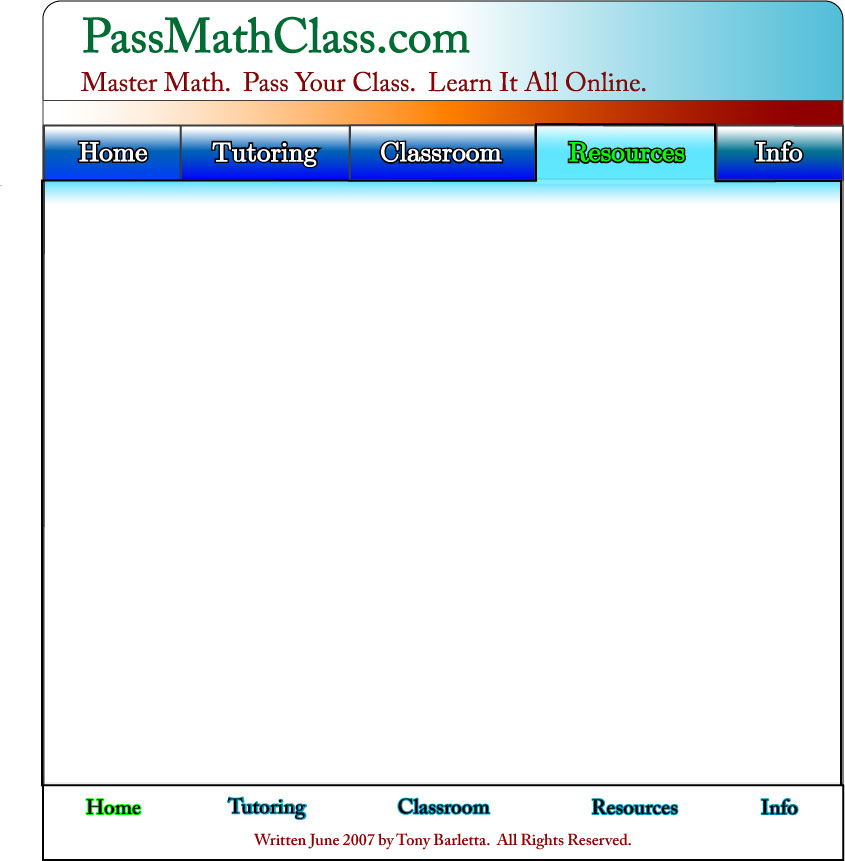This is a collection of free math lessons. This material was written by the founders of Pass Math Class to be unlike a textbook. We have seen many math textbooks that are great at rigorous proof and complex notation but terrible at actually explaining the material.

Feel free to go through all of the lessons, or just the ones you may need. And remember, we've got one-on-one tutoring AND drop-in math help.

We have left out the rigorous proofs and confusing vocabulary – we have kept the most simple essence of each concept.

Free Online Math LessonsFree Math Lessons
Calculus
Pre-Algebra
Algebra
Pre-Calculus